# KSEEB Solutions for Class 6 Maths Chapter 4 Basic Geometrical Ideas Ex 4.1

Students can Download Chapter 4 Basic Geometrical Ideas Ex 4.1 Questions and Answers, Notes Pdf, KSEEB Solutions for Class 6 Maths helps you to revise the complete Karnataka State Board Syllabus and score more marks in your examinations.

## Karnataka State Syllabus Class 6 Maths Chapter 4 Basic Geometrical Ideas Ex 4.1

Question 1.
Use the figure to name:
a) Five points
b) a line
c) Four rays
d) Five Line Segments
Solution: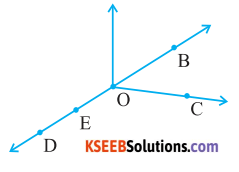a) The five points are D, E, O, B and C
b) $$\overrightarrow{\mathrm{BD}}$$
c) $$\overrightarrow{\mathrm{OD}}, \overrightarrow{\mathrm{OB}}, \overrightarrow{\mathrm{OC}}, \overrightarrow{\mathrm{OE}}$$
d) $$\overrightarrow{\mathrm{DE}}, \overrightarrow{\mathrm{EO}}, \overrightarrow{\mathrm{OB}}, \overrightarrow{\mathrm{BE}}, \overrightarrow{\mathrm{OC}}$$

Question 2.
Name the line given in all possible ( twelve) ways, choosing only two letters at a time from the four given.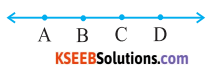Solution:
$$\overrightarrow{\mathrm{AB}}, \overrightarrow{\mathrm{BC}}, \overrightarrow{\mathrm{CD}}, \overrightarrow{\mathrm{BA}}, \overrightarrow{\mathrm{CB}}, \overrightarrow{\mathrm{DC}}, \overrightarrow{\mathrm{AD}}, \overrightarrow{\mathrm{DA}}, \overrightarrow{\mathrm{AC}}, \overrightarrow{\mathrm{CA}}, \overrightarrow{\mathrm{BD}}$$Question 3.
Use the figure to name:
a) Line containing point E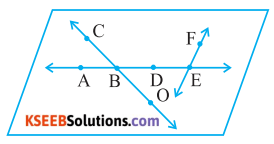b) Line passing through A.
c) Line on Which O lies.
d) Two pairs of intersecting lines
Solution:
a) $$\overleftrightarrow{AE}$$
b) $$\overleftrightarrow{AE}$$
c) $$\overleftrightarrow{OC}$$
d) $$\overleftrightarrow{OC}$$ and $$\overleftrightarrow{AE}$$, $$\overleftrightarrow{AE}$$ and $$\overleftrightarrow{EF}$$

Question 4.
How many lines can pass through
(a) one given point?
(b) two given points?
Solution:
a) infinite number of lines can pass through a single point.
b) Only one line can pass through two given pointsQuestion 5.
Draw a rough figure and label suitably in each of the following cases: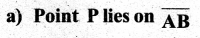b) $$\overleftrightarrow{XY}$$ and $$\overleftrightarrow{PQ}$$ intersect at M
c) Line l contains E and F but not D.
d) $$\overleftrightarrow{OP}$$ and $$\overleftrightarrow{OQ}$$ meet at O.
Solution: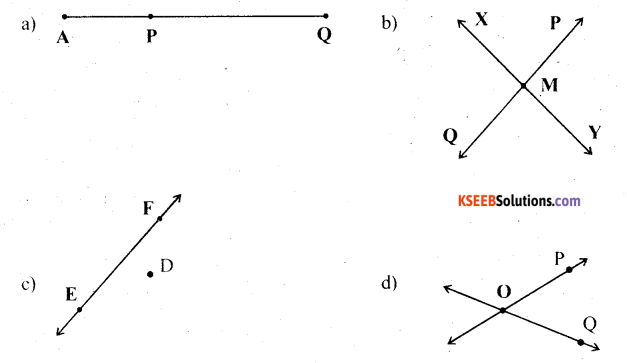Question 6.
Consider the following figure of line $$\overleftrightarrow{MN}$$. Say whether following statements are true or false in context of the given figure.
a) Q, M, O, N, P are points on the line $$\overleftrightarrow{MN}$$ – Truef) M is point on ray $$\overleftrightarrow{OP}$$ – False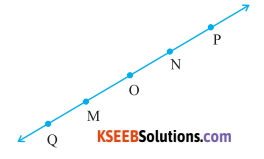g) Ray $$$\overleftrightarrow{OP}$$$ is different from ray $$\overleftrightarrow{QP}$$ – True
h) Ray $$\overleftrightarrow{OP}$$ same as ray $$\overrightarrow{\mathrm{OM}}$$ – Terue
i) Ray $$\overrightarrow{\mathrm{OM}}$$ is net opposite to ray $$\overleftrightarrow{OP}$$
j) O is net an initial point of $$\overleftrightarrow{OP}$$ – False
k) N is the initial point of $$\overrightarrow{\mathrm{NP}}$$ and $$\overrightarrow{\mathrm{NM}}$$ – True

error: Content is protected !!